Wednesday , March 27 2019

# Advanced Formula, Salesforce Platform unleashed!!!

### Introduction to formula fields and Advanced Formulas

Formula Field is a powerful capability that has been provided to us by force.com platform, it gives the ability to display the data according to our need. With the help of formula field we can perform calculations on numeric fields and display them as a percentage or if we want to convert a field into a clickable hyperlink or if we want to calculate number of days between two date fields of an object, we can accomplish all these tasks using formula fields.

To create a formula field, we need to understand the formula editor, the place where we create the logic of our formula field. To reach to formula editor we need to follow the following steps.

1. Go to a custom Object or standard object detail page over there scroll down to the section of custom fields.
2. Click on new button and select formula field give it a label and select the return type of the resulting value as shown below.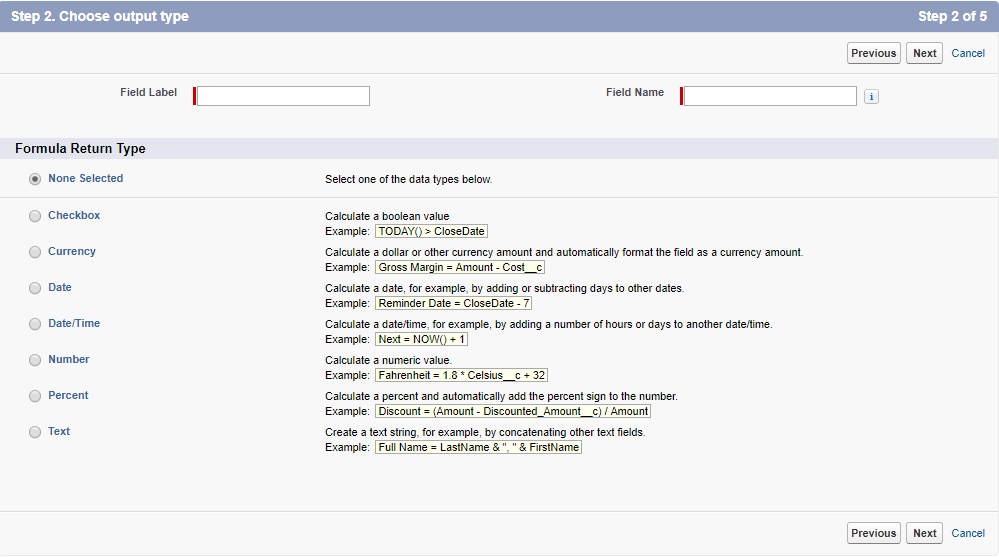3. After selecting the Return type you are directed to the screen where you can create your formula, i.e Formula Editor.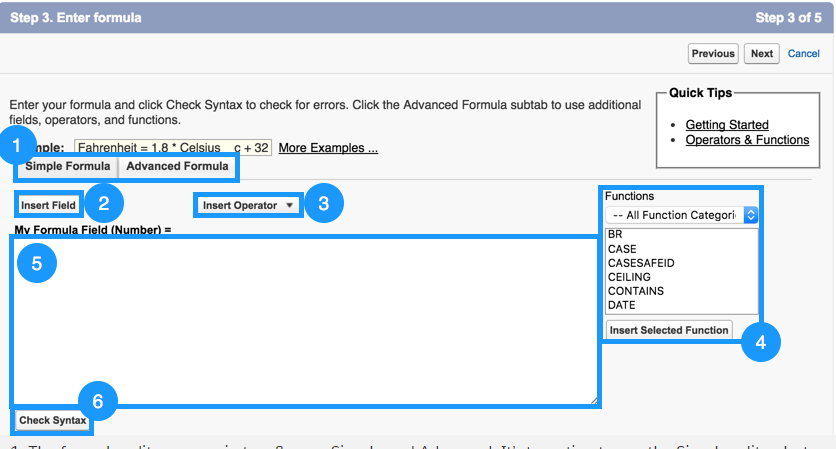4. Formula Editor comes in two version, Simple and advanced, the only difference is that the advanced version has more options.
5. Insert Field button in the editor provides point and click access to the fields of the std-Object/ Custom Object and to the fields of object related to this entity.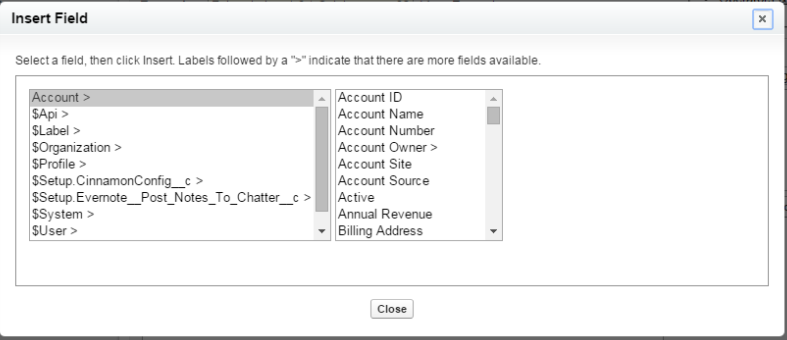6. Insert Operator button provides us with the point click way to put logical operators in the formula.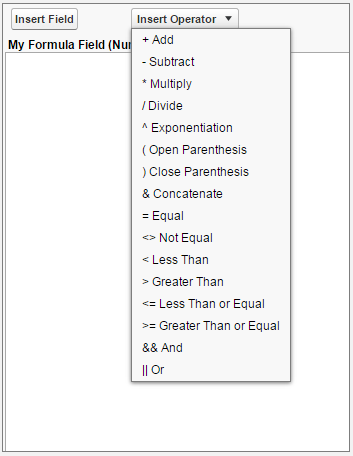7. Lastly the Function panel provides the ease of access to insert a function in the formula.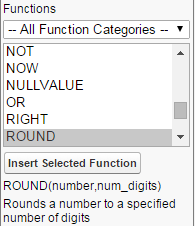This pretty much sums up the formula editor and from here we will dig deep into the formulas and the use case where we can implement some useful logics.

## Advanced Formula using Checkbox basic logic

### Checkbox Type in Formulas

Checkbox is a Boolean field which is either selected or not selected i.e. it is either true or false. A use case of this could be to check if an account has more than 500 employees or not, if the formula evaluates to be true then the large account checkbox will be checked and if it is false then the checkbox will be unchecked.

### Basic use of Logical Operators

Logical operators are adapted from the real world conversation i.e. if –this happens –then I will do this, and, or, not. We will discuss these operators in detail below:

### AND()

In this function at least two arguments are required, but it can handle more than that. This function will return true only if all the arguments are true. Schematic of this statement is shown below in the table.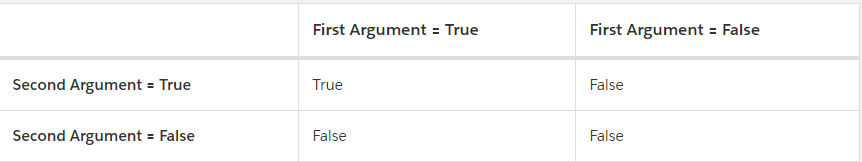Suppose you a sales rep wants to know whether to reach out to a contact via email or mobile phone or if a contact has opted out of email and mentioned Do Not Call, then we would like a checkbox to be checked, indicating to the sales rep that this contact wishes not to be contacted. In this case we will use AND () function and the argument we will check is Do not Contact. The formula in the editor would look something like as shown below.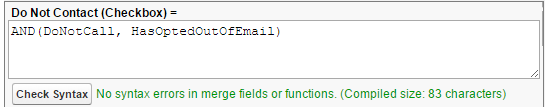If a contact has selected both emails opt out and do not call option then the third checkbox will be checked indicating that this contact should not be contacted, but in case if only one option is selected then this checkbox will not be checked.

### OR()

This function is similar to the And (), it also takes two variables as an argument, but the only difference is that, this function will return true if any one of the argument turns out to be true. Schematic of this statement is shown below in the table.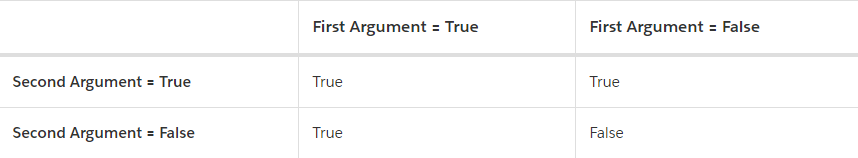Suppose on a contact object we want to a checkbox to be selected if the contact is the CEO or is at an executive level. To know if a contact satisfies the above mentioned requirements, we need to check the title of the contact i.e. it begins with “Chief” or the title contains “President” or it the title contains “Executive”.
To find these values in a text we would be required to use two different methods.
1. Begins(text, compare_text) this method compares the two strings and checks if the “text” begins with “compare_text”, if this condition evaluates to be true then it will return true else it will return false.
2. Contains(text, compare_text) this method compares the two strings and checks if the “text” contains the “compare_text”, if this condition evaluates to be true then it will return true else it will return false.
The resulting formula would look something like below: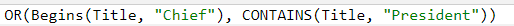Or we can also write them using the symbols as shown below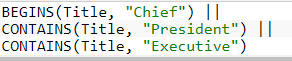Now whenever, if one of these conditions turn out to be true the checkbox will be checked.

### NOT()

The NOT() method inverses the Boolean value of any field i.e. it will change true to false or false to true. For this let’s take an example where we want a checkbox to be checked if all the important information of a record has been filled by the rep, for example, the formula would something as shown below.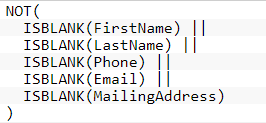Or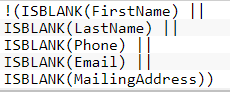### IF()

This method can be written as IF(Testing_Condition, If_True_Execute_This, Else_Execute_This), it takes three arguments. First a condition to test, this is the condition which defines the flow of the execution, of this condition evaluates to be true, then the flow is transferred to the second argument i.e. “If_True_Execute_This” if not then the flow is transferred to the third argument i.e. “Else_Execute_This”.

Let’s take an example where we first want to check if a contact is executive or not. If it turns out to be true then we would like to check its department, if the department is “sales” then we will check its area code which should be “212” else return false and if the department turns out to be “Marketing” then the area code should be “415”, else return false. This can be represented in a if Method as below.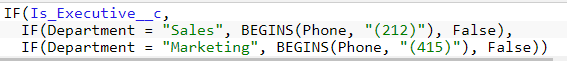So that’s how we use Checkbox with the formula field.

## Using Percentage, Number and Currency in the Formula Fields

To use number, currencies and percentage in formula field, we first need to understand the characteristic of each field. Below is the schematic of the three return types.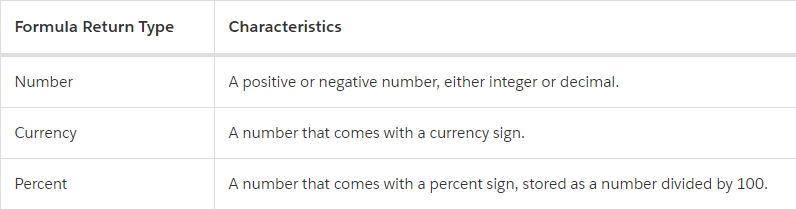Now we will discuss about some operators which are commonly used with these fields.

### Mathematical operators

Arithmetic operators like (+,-,*,/) are used to perform calculations on the numeric field values and these operations can be grouped in parenthesis as well. We can also use logical operators as well on these fields like (>,<,<=, >=, !=).
Formula editor also provide other Mathematical formulas like Round(), min(), max() etc.
Now let’s see how to use these return type in a formula field.

In this example our goal is to find Interest on an account, for this we first create a currency field on Account named Principal and it will have 2 decimal places. After we have created this field we will create another field “Interest Rate”, it would be a formula field with return type percent. This field is dependent on the amount present in the principal field and is evaluated as mentioned below.

Interest Rate equals to 0.02 if the amount in the principal is less than 10000, it is 0.03 if the principal is between 10000 and 100000, it 0.04 for values greater than 100000. To evaluate the value of interest rate we will make use of the following formula.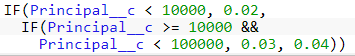Now to find the interest we have the principal and we have the interest rate as well.

To find the interest we make use of the formula A=Pe^(RT) where A is accumulated Interest on Account, P is the Principal, R is the rate of Interest and T is the number of year the account has been open and e is the mathematical constant.

To recreate this formula in Salesforce we need to implement another mathematical function provided in formula editor i.e. EXP(Num) this will raise the mathematical constant to the power of the Num.

We have already create a formula to find R and to find T we need to calculate this using another method in the formula editor that is Today() and we need to provide the value returned by this method to another Method Year(). This will return only the year of the present date. From this value we will subtract the year when the account was opened in the salesforce by using another method Value() which converts a text into integer. Your final formula should look something like this.

Principal__c * EXP(Interest_Rate__c * (YEAR(TODAY()) – VALUE(YearStarted)))

Now we will discuss about the Date and Date/Time Fields in formula

## Date and Date/Time fields in Formula

To understand how to use Date and Date/Time field in formula we need to first understand the two fields. A Date field holds year month and day values and Date/Time have an extra value of time along with the other values. The time value is stored as GMT but it is displayed as per the current organizations time zone.

Also consider that when subtracting two date values a whole number is returned and when two Date/Time values are subtracted a fraction value is returned. We have to build formula fields keeping these factors about Date and Date/Time field in mind.

### DateValue() and DateTimeValue()

Salesforce platform provides us with methods which help us in working with Date and Date/Time fields. To begin with if we want to convert a Date/Time value to Date we will use method DateValue(), it takes a text value or Date/Time and provides a Date as a return. Similarly we have DateTimeValue() method which returns a Date/Time Value.

### Today() and now()

These methods provide same information i.e. today’s date the only difference is that the value returned by Today() is a Date value and value returned by Now() is a Date/Time Value. To find the day, month, year of a Date value we use the methods day(), month(), year() respectively, these methods take a Date value as an argument and return a number as value corresponding to the argument.

It seems pretty straightforward to calculate on Date fields but things get complicated when we take business days into the consideration. Let’s have look at the example to understand in detail.

We want to create a formula field which adds 3 business days to today’s date. This seems simple If today’s date is a Sunday, Monday or Tuesday because adding 3 business days is similar to Today() + 3. But what if today is Wednesday, Thursday, Friday, adding business days here is equal to Today +3 +2 i.e. business days and the 2 weekend days. Also if Today is Saturday then again it is different than the previous two condition, it is equal to Today() + 3+ 1, i.e. only one weekend day.

To implement this logic we will make use of another method provided to us by the salesforce i.e. case(). This method takes and expression and compares it the n number of cases and transfer the flow of logic to the result value which is corresponding the case which matches the expression it’s syntax is as shown below.

Case(Expression, Case1, result1, case2, result2,……, else result)

To begin the implementation we first need to know which day is today, for this we need to find the number of days between today and a known past Sunday. After finding the number of days we would use another method MOD() and divide the number of days with 7 and the result of this method will give us the number corresponding to the current day i.e. 0 for Monday, 1, for Tuesday, 2 for Wednesday, 3 for Thursday etc. The final formula would look as shown below.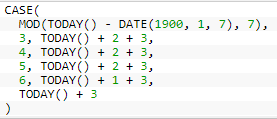Now we will learn about using Picklist in the formula fields

### Picklist in the Formula

Picklist is a field where we select a value from a drop down list which is populated with some predefined values. A formula field cannot return a picklist as a value but it can reference a value which is selected in a picklist.

### ISPICKVAL() and PRIORVALUE()

IsPickVal(picklistField, compare_text) method takes two arguments and returns true if the value of the picklist field matches that of the compare text. PriorValue(picklistField, compare_Text) method as the name suggests compares the previous value of the picklist to the compare text and returns true if they match.

Let’s have a look at the below scenario where Account.rating picklist value is used along with other parameters to define the priority of the case.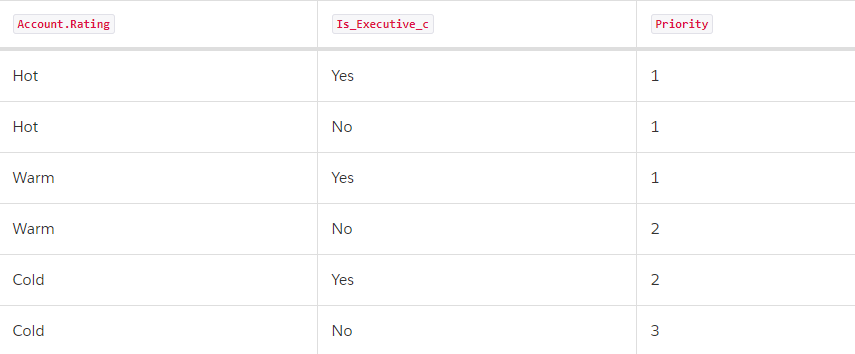The formula to implement the above logic would look similar to the below code.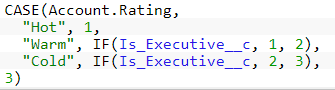Now we will discuss the use of text values in the formula.

## Use Text Formula

Text in formula field can be useful if we want to display two concatenated text fields or if we want to convert numbers, currency and dates to text or if we want to display text conditionally. For example FirstName & ” ” & LastName .

This will display first and last name along with a space between the two.

### Common functions to use with Text Return type in Formula

Salesforce platform provides us with many functions to make it easy to work with text values. With the help of these functions we can easily convert other values to text and vice versa or you can find a specific string in the text. Let’s dig deep in to these functions.

#### Text()

This method accepts one argument and will return a corresponding text value. It accepts any value i.e. number, percent, currency, date and date/time, picklist. The resulted text is not formatted i.e. no commas or currency symbol or percentage sign for e.g. if we give Text(Percent) then if the percent is equal to 30% it will return 0.3.

When the Text method receives a Date or Date/Time value it will convert it in to the corresponding Date and Date/Time with the standard formatting for e.g. Text(DateVar) will return 2017-09-28 for September 28th 2017, and Text(DateTimeVar) will return 2017-09-28 18:00:00Z for September 28th 2017 6 PM, the ‘Z’ in the end means that the value returned is in GMT. The result of the Date/Time will return the text in GMT only ir respective of the time zone of the org.

We have already used other string functions like Begin() and Contains(), there is one more String method which is used commonly i.e. Substitute(Text, oldString, newString) it will return the text and will replace any instance of the oldString with the newString. Let’s dig deep in using the text as a return type in formula field with the below use case.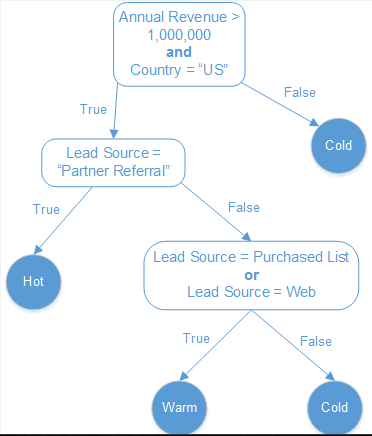In the above examples let’s write down the conditions:-

Hot—It refers to the lead with its Annual Revenue is more than a million, it’s Country is the United States, and the Lead Source is Partner Referral.

Warm— It refers to the lead with its Annual Revenue is more than a million, it’s Country is the United States, and the Lead Source is either Purchased List or Web.

Cold—The lead doesn’t satisfy any of the above conditions.

To reach this automation we will create a formula which would look something like this.Based on the above rating we create a field which is showing a conditional text, i.e. based on the formula the value of the rating field will change. Now based on the value of this rating field we can create another field which will display an image corresponding to the value of the rating field. For this, we will use Image method in the formula editor and with the help of the Case method, we can change the address of the image we use the formula below to show conditional images.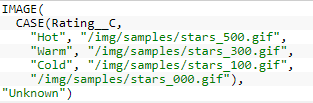Also Have a look at the below resources:

Also Have a look at the below learning resources:

### About Sumit DattaI am a 3x Certified Salesforce developer with overall 5 years of IT experience and 3 years of Implementation experience in Salesforce. I am here to share my knowledge and help Beginners in Salesforce to understand the concepts of Apex, Visualforce, Salesforce Lightning and Salesforce Configuration.

## How to use Jitterbit Cloud agent with Salesforce

How to use Jitterbit Cloud agent with Salesforce     Have you ever found yourself …

## How to create a Modal box in Lightning Component?

How to create a Modal box in Lightning Component? In this post we are going …

## How to send parameters in AuraEnabled methods in Salesforce Lightning Components?

How to send parameters in AuraEnabled methods in Salesforce Lightning Components? Passing value to and …

## How to use lightning accordion

How to use lightning accordion and how to dynamically add accordion section in lightning. In …